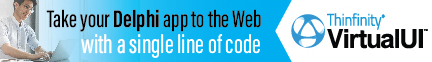DelphiBasicsDaySpanFunction Gives the fractional number of days between 2 dates DateUtils unit
 function DaySpan ( const ToDate, FromDate : TDateTime ) : Double;
Description
The DaySpan function subtracts the FromDate from the ToDate, returning the fractional days difference.

The Double return value contains the number of days as the integral part, and the part-day remainder as the fractional part.

For example, a difference of 2 days and 6 hours would give a value of 2.25
Related commands
 DaysBetween Gives the whole number of days between 2 dates DaysInAMonth Gives the number of days in a month DaysInAYear Gives the number of days in a year
Author linksDownload this web site as a Windows program.

 Example code : Find the days difference between two date+time values. var   fromdate, toDate : TDateTime; begin  // Set up our date variables   fromDate := EncodeDateTime(2000, 01, 01, 0, 0, 0, 0);   toDate   := EncodeDateTime(2000, 01, 02, 12, 0, 0, 0);   // Display these dates and the days between them   ShowMessage('From date = '+DateTimeToStr(fromDate));   ShowMessage('To   date = '+DateTimeToStr(toDate));   ShowMessage('Fractional days difference = '+               FloatToStr(DaySpan(toDate, fromDate))+' days'); end; Show full unit code From date = 01/01/2000   To   date = 02/01/2000 12:00:00   Fractional days difference = 1.5 days
 Delphi Programming © Neil Moffatt 2002 - 2020. All rights reserved.  |  Home Page